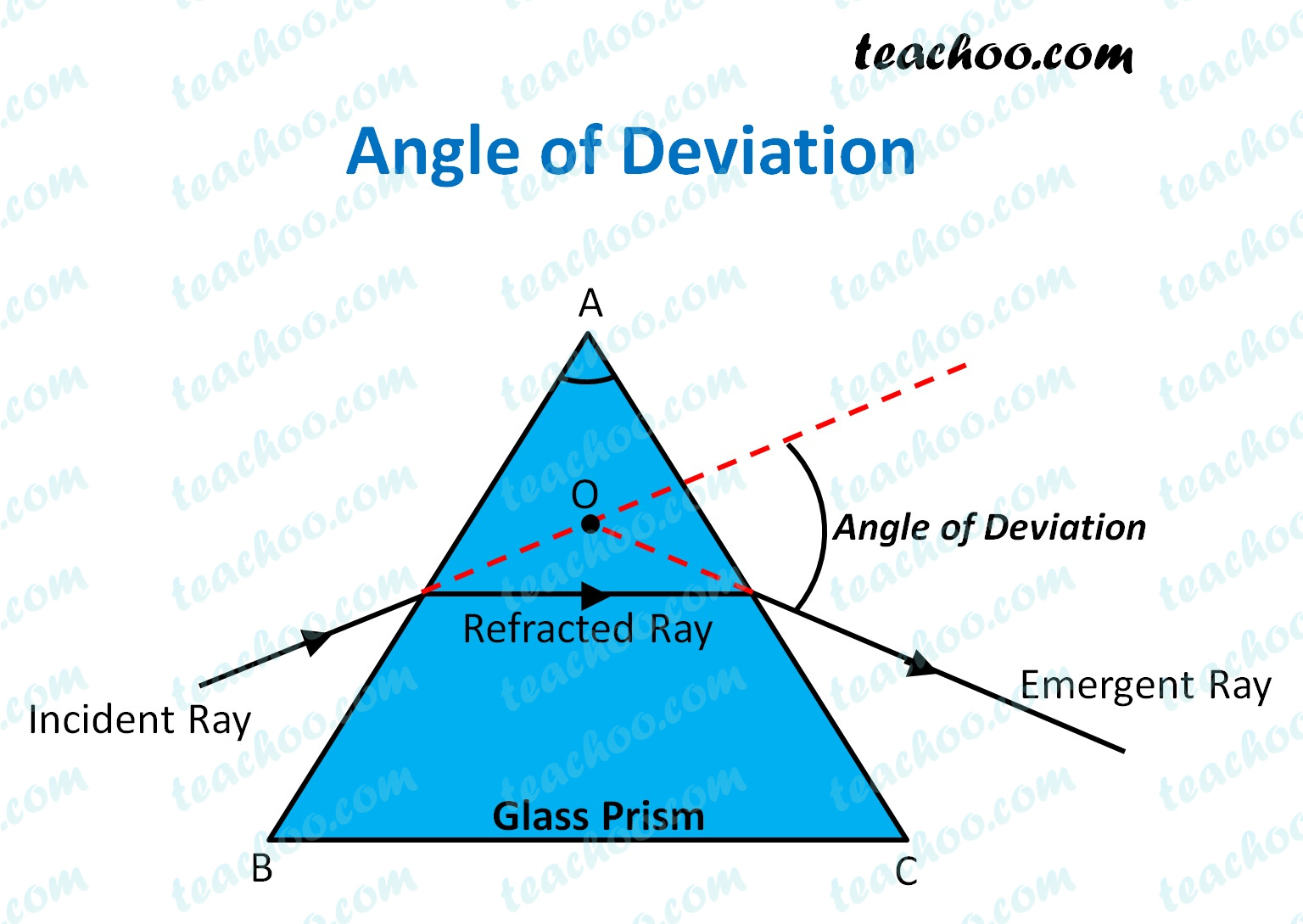Teachoo Questions

Class 10
Chapter 11 Class 10 - Human Eye and Colourful World

In a prism, the angle between the Incident Ray and the Emergent Ray is called the Angle of DeviationThe Angle of deviation in a prism depends upon:

• Refractive index of the prism -  The larger the refractive index, the larger the angle of deviation.
• Angle of the prism (i.e. Angle A in the figure) -  The larger the prism angle, the larger the deviation angle.
• Angle of incidence: Angle of deviation first increases with increasing incidence angle, and then decreases.

Learn in your speed, with individual attention - Teachoo Maths 1-on-1 Class